# 2020 seminars

## 07/01/2021, Thursday, 16:30–17:30 Europe/Lisbon Room P1, Mathematics Building — Online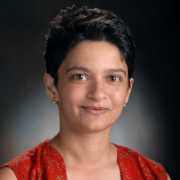Nilima Nigam, Simon Fraser University
Boundary integral strategies for the Steklov eigenproblem

In Steklov eigenproblems for elliptic operators, the spectral parameter links boundary traces of eigenfunctions to traces of the Neumann data. It is natural, therefore, to reformulate such eigenproblems in terms of boundary integral operators, which allow for nonsmooth boundaries. In this talk we describe such strategies in the context of Steklov problems for the Laplacian as well as the Helmholtz operator, and their use in studying questions arising in spectral geometry.

Please not that the talk will be at 16:30 Lisbon/London time.

Upcoming talks:

14 Jan - Serena Dipierro & Enrico Valdinoci, University of Western Australia

21 Jan - Charles Collot, Cergy Paris Université

28 Jan - Enrico Serra, Politecnico di Torino

## 14/01/2021, Thursday, 10:00–12:00 Europe/Lisbon OnlineSerena Dipierro & Enrico Valdinoci, University of Western Australia
Nonlocal Minimal Surfaces: interior regularity, boundary behavior and stickiness phenomena

Surfaces which minimize a nonlocal perimeter functional exhibit quite different behaviors than the ones minimizing the classical perimeter. We will investigate some structural properties of nonlocal minimal surfaces both in the interior of a given domain and in the vicinity of its boundary.

Among these peculiar features, an interesting property, which is also in contrast with the pattern produced by the solutions of linear equations, is given by the capacity, and the strong tendency, of adhering at the boundary. We will also discuss this phenomenon and present some recent results.

(These are two consecutive talks: Part I is given by Serena Dipierro, Part II by Enrico Valdinoci)

### Video

Please note that the seminar takes place at 10am Lisbon/London time, not the usual time for Lisbon WADE

## 21/01/2021, Thursday, 14:00–15:00 Europe/Lisbon Online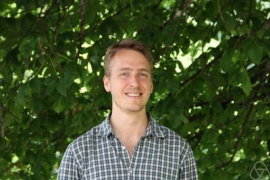Charles Collot, Cergy Paris Université
On the stability of equilibria for infinitely many particles

We study the evolution of a system of particles. Instead of the usual Hartree equation for density matrices, we consider the following equivalent model, proposed by de Suzzoni, of a Hartree type equation but for a random field:$$iX_t=-\Delta X +(w*\mathbb E(|X|^2))X.$$Above, $X:[0,T]\times \mathbb R^d\times \Omega$ is a time-dependent random field, $w$ a pair interaction potential, $*$ the convolution product and $\mathbb E$ the expectation. This equation admits equilibria which are random Gaussian fields whose laws are invariant by time and space translations. They are hence not localised and represent an infinite number of particles. We give a stability result under certain hypotheses, by showing that small perturbations scatter as $t\rightarrow \pm \infty$ to linear waves. This is joint work with de Suzzoni.

Please note that the link has changed.

## 28/01/2021, Thursday, 14:00–15:00 Europe/Lisbon OnlineEnrico Serra, Politecnico di Torino
NLS ground states on metric trees: existence results and open questions

We consider the minimization of the NLS energy on a metric tree, either rooted or unrooted, subject to a mass constraint. With respect to the same problem on other types of metric graphs, several new features appear, such as the existence of minimizers with positive energy, and the emergence of unexpected threshold phenomena. We also study the problem with a radial symmetry constraint that is in principle different from the free problem due to the failure of the Polya-Szego inequality for radial rearrangements. A key role is played by a new Poincaré inequality with remainder.

## 04/02/2021, Thursday, 14:00–15:00 Europe/Lisbon OnlineYvan Martel, École Polytechnique
On the asymptotic stability of kinks for $(1+1)$-scalar field models

The talk concerns stability properties of kinks for (1+1)-dimensional nonlinear scalar field models of the form $\partial_t^2 \phi - \partial_x^2 \phi + W'(\phi) = 0 \quad (t,x) \in {\bf \mathbb R}\times {\mathbb R}.$ We establish a simple and explicit sufficient condition on the potential $W$ for the asymptotic stability of a given moving or standing kink. We present applications of the criterion to some models of the Physics literature.

Work in collaboration with Michał Kowalczyk, Claudio Muñoz and Hanne Van Den Bosch.

See also the related work with Michał Kowalczyk and Claudio Muñoz.

## 11/02/2021, Thursday, 14:00–15:00 Europe/Lisbon OnlineHarbir Antil, George Mason University
Fractional PDEs: Control, Numerics, and Applications

Fractional calculus and its application to anomalous diffusion has recently received a tremendous amount of attention. In complex/heterogeneous material mediums, the long-range correlations or hereditary material properties are presumed to be the cause of such anomalous behavior. Owing to the revival of fractional calculus, these effects are now conveniently modeled by fractional-order differential operators and the governing equations are reformulated accordingly. Similarly, the potential of fractional operators has been harnessed in various scientific domains like geophysical electromagnetics, imaging science, deep learning, etc.

In this talk, fractional operators will be introduced and both linear and nonlinear, fractional-order differential equations will be discussed. New notions of optimal control and optimization under uncertainty will be presented. Several applications from geophysics, imaging science, and deep learning will be presented.

## 18/02/2021, Thursday, 14:00–15:00 Europe/Lisbon Online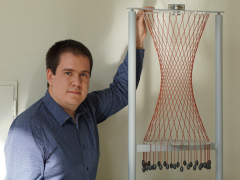The quasistatic limit is a convenient approximation in the modelling of several (suitable) mechanical systems, when the evolution occurs at a sufficiently slow time-scale. In this talk we discuss the validity of the quasistatic approximation in finite-dimensional rate-independent systems via a vanishing-inertia asymptotic analysis of dynamic evolutions. More precisely, we show the uniform convergence of dynamic solutions to the quasistatic one, employing the concept of energetic solution. Our work is motivated by the application to a family of models for biological and bio-inspired crawling locomotion. Hence a part of the seminar will focus on modelling: we will discuss how soft crawlers can be effectively described in our theoretical framework and briefly survey the relevance, or lack thereof, of inertia in some locomotion strategies. By a technical point of view, our application requires time-dependence of the dissipation potential and translation invariance of the potential energy.

## 25/02/2021, Thursday, 14:00–15:00 Europe/Lisbon OnlineIn this joint work with P. Souplet we develop a new, unified approach to the following two classical questions on elliptic PDE:

(i) the strong maximum principle for equations with non-Lipschitz nonlinearities; and

(ii) the at most exponential decay of solutions in the whole space or exterior domains.

Our results apply to divergence and nondivergence operators with locally unbounded lower-order coefficients, in a number of situations where all previous results required bounded ingredients. Our approach, which allows for relatively simple and short proofs, is based on a (weak) Harnack inequality with optimal dependence of the constants in the lower-order terms of the equation and the size of the domain, which we establish.

## 04/03/2021, Thursday, 14:00–15:00 Europe/Lisbon OnlineBozhidar Velichkov, Università di Pisa
Vectorial free boundary problems

The vectorial Bernoulli problem is a variational free boundary problem involving the Dirichlet energy of a vector-valued function and the measure of its support. It is the vectorial counterpart of the classical one-phase Bernoulli problem, which was first studied by Alt and Caffarelli in 1981.

In this talk, we will discuss some results on the regularity of the vectorial free boundaries obtained in the last years by Caffarelli-Shahgholian-Yeressian, Kriventsov-Lin, Mazzoleni-Terracini-V., and Spolaor-V.. Finally, we will present some new results on the rectifiability of the singular set obtained in collaboration with Guido De Philippis, Max Engelstein and Luca Spolaor.

## 04/03/2021, Thursday, 15:00–16:00 Europe/Lisbon OnlineDario Mazzoleni, Università di Pavia
Regularity of the optimal sets for the second Dirichlet eigenvalue

First of all, we recall the basic notions and results concerning shape optimization problems for the eigenvalues of the Dirichlet Laplacian.

Then we focus on the study of the regularity of the optimal shapes and on the link with the regularity of related free boundary problems.

The main topic of the talk is the regularity of the optimal sets for a "degenerate" functional, namely the second Dirichlet eigenvalue in a box. Given $D\subset \mathbb{R}^d$ an open and bounded set of class $C^{1,1}$, we consider the following shape optimization problem, for $\Lambda>0$,\begin{equation}\label{eq:main}\min{\Big\{\lambda_2(A)+\Lambda |A| : A\subset D,\text{ open}\Big\}},\end{equation}where $\lambda_2(A)$ denotes the second eigenvalue of the Dirichlet Laplacian on $A$.

In this talk we show that any optimal set $\Omega$ for $\eqref{eq:main}$ is equivalent to the union of two disjoint open sets, $\Omega^\pm$, which are $C^{1,\alpha}$ regular up to a (possibly empty) closed singular set of Hausdorff dimension at most $d-5$, which is contained in the one-phase free boundaries.

In particular, we are able to prove that the set of two-phase points, that is, $\partial \Omega^+\cap \partial \Omega^-\cap D$, is contained in the regular set.

This is a joint work with Baptiste Trey and Bozhidar Velichkov.

## 11/03/2021, Thursday, 14:00–15:00 Europe/Lisbon OnlineBruno Premoselli, Université Libre de Bruxelles
Towers of bubbles for Yamabe-type equations in dimensions larger than 7

In this talk we consider perturbations of Yamabe-type equations on closed Riemannian manifolds. In dimensions larger than 7 and on locally conformally flat manifolds we construct blowing-up solutions that behave like towers of bubbles concentrating at a critical point of the mass function. Our result does not assume any symmetry on the underlying manifold.

We perform our construction by combining finite-dimensional reduction methods with a linear blow-up analysis in order to sharply control the remainder of the construction in strong spaces. Our approach works both in the positive and sign-changing case. As an application we prove the existence, on a generic bounded open set of $\mathbb{R}^n$, of blowing-up solutions of the Brézis-Nirenberg equation that behave like towers of bubbles of alternating signs.

## 18/03/2021, Thursday, 14:00–15:00 Europe/Lisbon OnlineGabriele Benomio, Princeton University
A new instability for higher dimensional black holes

The dynamics of solutions to the Einstein equations is richer in dimensions higher than $3+1$. In contrast with the classical stability of stationary, asymptotically flat black hole solutions in $3+1$ dimensions, some families of higher dimensional black holes suffer from dynamical instabilities. I will discuss a subtle instability affecting a wide class of higher dimensional black holes which has not been previously observed in the literature. This new instability is, in a sense, more fundamental than the other known instability phenomena in higher dimensions and can be related to a precise geometric property of the class of spacetimes considered.

## 18/03/2021, Thursday, 15:00–16:00 Europe/Lisbon OnlineShrish Parmeshwar, University of Bath
Global-in-Time Solutions to the N-Body Euler-Poisson System

We investigate the $N$-Body compressible Euler-Poisson system, modelling multiple stars interacting with each other via Newtonian gravity. If we prescribe initial data so that each star expands indefinitely, one might expect that two of them will collide in finite time due to their expansion, and the influence of gravity. In this talk we show that there exists a large family of initial positions and velocities for the system such that each star can expand for all time, but no two will touch in finite time. To do this we use scaling mechanisms present in the compressible Euler system, and a careful analysis of how the gravitational interaction between stars affects their dynamics.

## 25/03/2021, Thursday, 11:00–12:00 Europe/Lisbon OnlineYukihiko Nakata, Aoyama Gakuin University, Tokyo
Period two solution for a class of distributed delay differential equations

We consider a periodic solution for a class of distributed delay differential equations.

A period two solution for distributed delay differential equations, where the period is twice the maximum delay, is shown to satisfy a Hamiltonian system of ordinary differential equations, from which we can construct the period two solution for the distributed delay differential equation.

The idea is based on Kaplan & Yorke (1974, JMAA) for a discrete delay differential equation. We present distributed delay differential equations that have periodic solutions expressed in terms of the Jacobi elliptic functions.

**Note that the link is not the usual one.**

## 01/04/2021, Thursday, 14:00–15:00 Europe/Lisbon OnlineAdditive manufacturing (or 3-d printing) is a new exciting way of building structures without any restriction on their topologies. However, it comes with its own difficulties or new issues. Therefore, it is a source of many interesting new problems for optimization. I shall discuss two of them and propose solutions to these problems, but there is still a lot of room for improvement!

First, additive manufacturing technologies are able to build finely graded microstructures (called lattice materials). Their optimization is therefore an important issue but also an opportunity for the resurrection of the homogenization method ! Indeed, homogenization is the right technique to deal with microstructured materials where anisotropy plays a key role, a feature which is absent from more popular methods, like SIMP. I will describe recent work on the topology optimization of these lattice materials, based on a combination of homogenization theory and geometrical methods for the overall deformation of the lattice grid.

Second, additive manufacturing, especially in its powder bed fusion technique, is a very slow process because a laser beam must travel along a trajectory, which covers the entire structure, to melt the powder. Therefore, the optimization of the laser path is an important issue. Not only do we propose an optimization strategy for the laser path, but we couple it with the usual shape and topology optimization of the structure. Numerical results show that these two optimizations are tightly coupled.

This is a joint work with many colleagues, including two former PhD students, P. Geoffroy-Donders and M. Boissier.

## 08/04/2021, Thursday, 14:00–15:00 Europe/Lisbon OnlineExtensions operators are a classical tool to provide uniform estimates and gain compactness in the homogenization of integral functionals over perforated domains. In this talk we discuss the case of non-local functionals. The results are obtained in collaboration with Andrea Braides and Lorenza D'Elia.

## 15/04/2021, Thursday, 14:00–15:00 Europe/Lisbon OnlineNicola Fusco, Università di Napoli "Federico II"
Asymptotic stability for the gradient flow of some nonlocal energies

I will start by discussing some recent results on the asymptotic stability of the $H^{-1}$-gradient flow of the perimeter, the so called surface diffusion. Then I will consider the $H^{-1}$-gradient flow of some energy functionals given by the area of an interface plus a non local volume term. This is a joint work with E. Acerbi, V. Julin and M. Morini

## 15/04/2021, Thursday, 15:00–16:00 Europe/Lisbon Online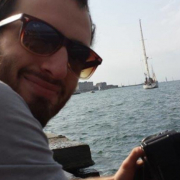Riccardo Scala, Università degli Studi di Siena
Nonlocality features of the area functional and the Plateau problem

We briefly discuss the definition of relaxation of the area functional. The relaxed area functional, denoted by $A$, extends the classical area functional, which, for any "regular" map $v:U\subset \mathbb{R}^n\rightarrow \mathbb{R}^N$ evaluates the $n$-dimensional area of its graph over $U$. The problem of determining the domain and the expression of $A$ is open in codimension greater than 1. Specifically, this relaxed functional turns out to be nonlocal and cannot be expressed by an integral formula. We discuss how it is related to classical and nonclassical versions of the Plateau problem. As a main example, we try to understand what is the relaxed graph of the function $x/|x|$, a question that surprisingly remained open for decades.

## 22/04/2021, Thursday, 14:00–15:00 Europe/Lisbon OnlineJuan Luis Vázquez, Universidad Autónoma de Madrid
The theory of fractional $p$-Laplacian equations

We consider the time-dependent fractional $p$-Laplacian equation with parameter $p\gt 1$ and fractional exponent $0\lt s\lt 1$. It is the gradient flow corresponding to the Gagliardo fractional energy. Our main result is the asymptotic behavior of solutions posed in the whole Euclidean space, which is given by a kind of Barenblatt solution whose existence relies on delicate analysis. We will concentrate on the sublinear or “fast” regime, $1\lt p \lt 2$, since it offers a richer theory. Fine bounds in the form of global Harnack inequalities are obtained as well as solutions having strong point singularities (Very Singular Solutions) that exist for a very special parameter interval. They are related to fractional elliptic problems of nonlinear eigenvalue form. Extinction phenomena are discussed.

## 29/04/2021, Thursday, 14:00–15:00 Europe/Lisbon OnlineMany stochastic systems can be viewed as gradient flow ('steepest descent') in the space of probability measures, where the driving functional is a relative entropy and the relevant geometry is described by a dynamical optimal transport problem. In this talk we focus on these optimal transport problems and describe recent work on the limit passage from discrete to continuous.

Surprisingly, it turns out that discrete transport metrics may fail to converge to the expected limit, even when the associated gradient flows converge. We will illustrate this phenomenon in examples and present a recent homogenisation result.

This talk is based on joint work with Peter Gladbach, Eva Kopfer, and Lorenzo Portinale.

## 29/04/2021, Thursday, 15:00–16:00 Europe/Lisbon OnlineMax Fathi, Université de Paris
A proof of the Caffarelli contraction theorem via entropic interpolation

The Caffarelli contraction theorem states that optimal transport maps (for the quadratic cost) from a Gaussian measure onto measures that satisfy certain convexity properties are globally Lipschitz, with a dimension-free estimate. It has found many applications in probability, such as concentration and functional inequalities. In this talk, I will present an alternative proof, using entropic interpolation and variational arguments. Joint work with Nathael Gozlan and Maxime Prod'homme.

## 06/05/2021, Thursday, 14:00–15:00 Europe/Lisbon OnlineThe Dysthe equation is a higher order approximation of the water waves system in the modulation (Schrödinger) regime and in the infinite depth case. After reviewing the derivation of the Dysthe and related equations, we will focus on the initial-value problem. We prove a small data global well-posedness and scattering result in the critical space $L^2(\mathbb R^2)$. This result is sharp in view of the fact that the flow map cannot be $C^3$ continuous below $L^2(\mathbb R^2)$.

Our analysis relies on linear and bilinear Strichartz estimates in the context of the Fourier restriction norm method. Moreover, since we are at a critical level, we need to work in the framework of the atomic space $U^2_S$ and its dual $V^2_S$ of square bounded variation functions.

We also prove that the initial-value problem is locally well-posed in $H^s(\mathbb R^2)$, $s\gt 0$.

Our results extend to the finite depth version of the Dysthe equation.

This talk is based on a joint work with Razvan Mosincat (University of Bergen) and Jean-Claude Saut (Université Paris-Saclay).

## 13/05/2021, Thursday, 14:00–15:00 Europe/Lisbon OnlineThe use of the entropic regularization and the relative Sinkhorn algorithm in order to calculate optimal transport problems is nowadays widely used.

We propose a new viewpoint on application of this regularization technique to variational mean-field games with quadratic Hamiltonian. In particular we focus on the fact that this regularization amounts to solving second order variational mean-field games, dropping in particular the requirement for the regularization parameter to be small, whenever we are interested in the case with diffusion.

Based on a joint work with J.-D. Benamou, G. Carlier and L. Nenna.

## 20/05/2021, Thursday, 15:00–16:00 Europe/Lisbon OnlineMariana Smit Vega Garcia, Western Washington University
Regularity of almost minimizers with free boundary

We study almost minimizer for functionals that yield a free boundary, as in the work of Alt-Caffarelli and Alt-Caffarelli-Friedman. The almost minimizing property can be understood as the defining characteristic of a minimizer in a problem that explicitly takes noise into account. In this talk, we discuss the regularity of almost minimizers to energy functionals with variable coefficients. This is joint work with Guy David, Max Engelstein & Tatiana Toro.

** Please note that the seminar is one hour later than the usual time **

## 27/05/2021, Thursday, 14:00–15:00 Europe/Lisbon OnlineJulio D. Rossi, Universidad de Buenos Aires
Non Linear Mean Value Properties for Monge-Ampère Equations

In recent years there has been an increasing interest in whether a mean value property, known to characterize harmonic functions, can be extended in some weak form to solutions of nonlinear equations. This question has been partially motivated by the surprising connection between Random Tug-of-War games and the normalized $p-$Laplacian discovered some years ago, where a nonlinear asymptotic mean value property for solutions of a PDE is related to a dynamic programming principle for an appropriate game.

Our goal in this talk is to show that an asymptotic nonlinear mean value formula holds for the classical Monge-Ampère equation.

Joint work with P. Blanc (Jyvaskyla), F. Charro (Detroit), and J.J. Manfredi (Pittsburgh).

## 30/09/2021, Thursday, 14:00–15:00 Europe/Lisbon OnlineRafael D. Benguria, Universidad Católica de Chile
Gagliardo-Nirenberg-Sobolev Inequalities and their counterparts on bounded domains

The Gagliardo-Nirenberg-Sobolev (GNS) inequalities have played a major role in applied mathematics and mathematical physics in more than half a century. In this talk I will present several new results concerning the counterparts of the GNS inequalities on bounded domains. Of course concentration of the minimizers of the GNS inequalities is a main tool in the proof of existence of minimizers on bounded domains. Naturally concentration occurs in the interior for Dirichlet boundary conditions and on the boundary for Neumann boundary conditions. In the Neumann case this leaves a set of interesting open problems depending on the characteristic of the boundary. This is in part joint work with Cristobal Vallejos (Penn State U.) and Hanne Van Den Bosch (U. de Chile) and in part with Soledad Benguria (U. Wisconsin, Madison).## 07/10/2021, Thursday, 14:00–15:00 Europe/Lisbon OnlineMonica Clapp, Universidad Nacional Autónoma de México
Optimal partitions for the Yamabe equation

The Yamabe equation on a Riemannian manifold $(M,g)$ is relevant to the question of finding a constant scalar curvature metric on $M$ that is conformally equivalent to the given one.

An optimal $\ell$-partition for the Yamabe equation is a cover of $M$ by $\ell$ pairwise disjoint open subsets such that the Yamabe equation with Dirichlet boundary condition has a least energy solution on each one of these sets, and the sum of the energies of these solutions is minimal.

We will present some recent results obtained in collaboration with Angela Pistoia (La Sapienza Università di Roma) and Hugo Tavares (Universidade de Lisboa) that establish the existence and qualitative properties of such partitions.

If time allows, we will also present some results on symmetric optimal partitions obtained in collaboration with Angela Pistoia, and with Alberto Saldaña (UNAM) and Andrzej Szulkin (Stockholm University).

## 14/10/2021, Thursday, 16:00–17:00 Europe/Lisbon Online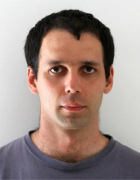Juraj Földes, University of Virginia
Stochastic approach to boundary regularity of hypoelliptic PDEs

We will discuss the almost sure behavior of solutions of stochastic differential equations(SDEs) as time goes to zero. Our main general result establishes a functional law of the iterated logarithm (LIL) that applies in the setting of SDEs with degenerate noise satisfying the weak Hormander condition. We will introduce large deviations to provide some details of proofs. Furthermore, we apply the stochastic results to the problem of identifying regular points for hypoelliptic diffusions and obtain criteria for well posedness of degenerate equations.

This is a joint work with David Herzog and Marco Carfagnini

## 21/10/2021, Thursday, 14:00–15:00 Europe/Lisbon OnlineSimone Dovetta, Università degli Studi di Roma "La Sapienza"
Action versus energy ground states in nonlinear Schrödinger equations

The talk investigates the relations between normalized critical points of the nonlinear Schrödinger energy functional and critical points of the corresponding action functional on the associated Nehari manifold.

First, we show that the ground state levels are strongly related by the following duality result: the (negative) energy ground state level is the Legendre—Fenchel transform of the action ground state level. Furthermore, whenever an energy ground state exists at a certain frequency, then all action ground states with that frequency have the same mass and are energy ground states too. We see that the converse is in general false and that the action ground state level may fail to be convex. Next we analyze the differentiability of the ground state action level and we provide an explicit expression involving the mass of action ground states. Finally we show that similar results hold also for local minimizers, and we exhibit examples of domains where our results apply.

The matter of the talk refers to joint works with Enrico Serra and Paolo Tilli.

## 04/11/2021, Thursday, 14:00–15:00 Europe/Lisbon OnlineKelei Wang, Wuhan University
Regularity of transition layers in Allen-Cahn equation

In this talk I will survey the regularity theory for transition layers in singularly perturbed Allen-Cahn equation, from zeroth order regularity to second order one. Some applications of this regularity theory will also be discussed, including De Giorgi conjecture, classification of finite Morse index solutions and construction of minimal hypersurfaces by Allen-Cahn approximation.

## 18/11/2021, Thursday, 14:00–15:00 Europe/Lisbon OnlineGiovanni Bellettini, ICTP and Università di Siena
On a conjecture of De Giorgi on the first variation of the Modica-Mortola functional

We shall discuss some (not so recent) results on a 1991 conjecture of De Giorgi concerning the Gamma limit of the square norm of the first variation of the Modica-Mortola functionals, and its relation with the Willmore functional.